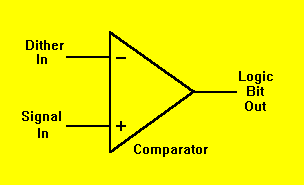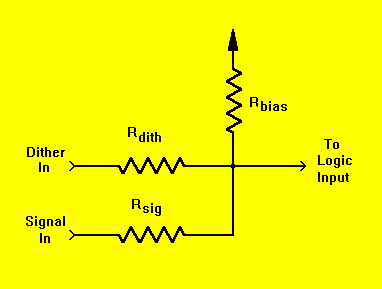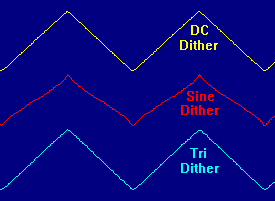Daqarta
Data AcQuisition And Real-Time Analysis
Scope - Spectrum - Spectrogram - Signal Generator
Software for Windows# Dither Tricks 2:

### by Bob Masta Interstellar Research

Previously, we looked at the use of dither with synchronous averaging to increase the resolution of an analog-to-digital Converter (ADC). Where the intrinsic noise in the signal was inadequate to provide self-dither, a separate DC or continuous wave dither source provided superior results, yielding increased ADC resolution without adding noise.

The natural question at this point is "how far can this go?" Can you keep reducing the number of physical ADC bits and recovering the resolution via dither plus averaging? As it turns out, the limiting case is a single-bit ADC, which requires nothing more than a simple level comparator. The idealized setup is shown in Figure 1:Fig. 1: An ideal single-bit ADC

The comparator output is high whenever the instantaneous signal is greater than the instantaneous dither, and low when it's less. Thus, as the signal swings more positive, the output is high a greater proportion of the time, and vice-versa. The output is essentially a pulse width modulated representation of the signal, such that the true value of the signal can be recovered by averaging.

Sampling consists of merely reading the digital output of the comparator at some specified rate. If the input signal is a repeating waveform, either a continuous wave or a transient such as an impulse response, then multiple repetitions of the signal must be synchronously averaged to recover the true waveform. If the input is effectively a DC level, then adjacent samples may be averaged over one or more dither cycles, using ordinary "moving window" averaging.

Watch That Comparator
It's important to note that the dither must swing over the entire input range; in fact it actually determines the input range. If the signal swing goes above or below the dither range, then the comparator output will be stuck high or low for as long as that condition persists, and the averaged output will be clipped. Conversely, if the dither range is greater than the signal range, the pulse width modulation will be reduced. So, as strange as it seems, you can actually control the ADC range by changing the dither level.

The comparator function can often be approximated by an ordinary logic gate input, with excellent results. Figure 2 shows how the single-bit ADC can be simplified down to just a few resistors. The operation is functionally the same as for the comparator circuit: At higher signal levels, the dither will drive the logic input above threshold more often, and vice-versa.Fig. 2: A logic-input single-bit ADC

The dither and signal input resistors Rdith and Rsig provide range scaling. Together with the bias resistor Rbias they must be selected such that with the input signal at zero, the dither produces a 50 percent average duty cycle from the output of the logic gate. Usually, that means that with the dither also at zero, the input should be just at the logic gate threshold. For unipolar signals, the bias resistor is selected to give a 50 percent duty cycle when the input is at mid-scale, instead of zero. Alternatively, the dither may be unipolar with a bipolar signal. Or both may be unipolar, in which case one or the other must be limited to the zero-to-threshold range, and no bias resistor is used.

A simpler way to look at this may be to consider the two inputs to act as a sort of seesaw that pivots about the logic threshold: When the signal is at one end of its range, the dither must be at the opposite end of its range to just achieve threshold. The bias resistor simply provides an offset adjustment. Without the bias, if you ground the signal input you'll have an ordinary voltage divider acting on the dither input. When you then unground the signal (at the "bottom" of the divider), that voltage source can be seen to simply add to the divider output voltage. If you reverse the dither and signal positions, the analysis is the same.

A Working Example
As an example, this method was used with an ordinary PC printer port. The signal range was 2.5 V, and the dither range was 0 to 5 V. The signal and dither input resistors were 20 kohms each, and the -Error input (active low; pin 15) was used as the "comparator" logic input. An 82 kohm bias resistor was wired to the -Select input (active low; pin 17), which is an open collector output with a pull-up to the positive rail. Busy (pin 11) was used as an external trigger input from the signal sync (not shown).

Data collection was via the author's Daqarta for DOS software using the LPTX driver on a 486DX2-50 laptop. The input signal was a 1 kHz triangular wave, and the sample rate was set to 132 kHz. (Since a 1-bit "conversion" only requires reading a single port, it can be rather fast.) The waveforms shown in Figure 3 were obtained by averaging 256 frames, each of which was 512 samples (3.9 msec) in duration. An entire average took only a few seconds at this sample rate. (Note that only the first portion of each averaged trace is shown here.)The top trace (yellow) shows the effect of DC dither. As discussed last time, this was provided by a separate digital-to-analog Converter (DAC) whose value was incremented by the averager after every frame. (Here the printer data output port drove a simple R-2R ladder, whose output impedance was included in Rdith.) After all 256 frames, the DAC output had traversed the entire dither range. Since each frame was collected with a constant DC bias, no artifacts or distortion resulted.

The bottom trace (light blue) used a standard function generator to supply a triangular dither. Offset and level controls were adjusted to get a 0 to 5 V range. After a few quick tests, a frequency of about 11 Hz was found to give acceptably low artifacts and distortion.

The center trace (red) used exactly the same setup, but with the function generator set to give a sine wave at the same peak-to-peak level and offset. Since it traversed the same range as the triangle and the DC ramp, but not linearly, there is obvious distortion and compression in the recovered signal triangle waveform. However, the central portion is fairly linear, so a sinusoidal dither waveform could be used by keeping the input signal within that linear range.

For precision DC work, note that the logic threshold may not be constant over time or temperature, so some form of self-calibration may be needed for measuring DC values. For transient or evoked-response measurements the absolute DC level is often of no concern, only the response relative to baseline. In these cases you can just set the range a little larger to allow for DC drift.

To record continuous, non-repetitive signals you must average adjacent samples using conventional averaging, not the synchronous averaging we discussed previously. For example, to get 8-bit resolution (one part in 256), you would need to average 256 adjacent samples, using a triangular or ramp dither source which traversed its full range in this interval. The result of the average would be one 8-bit sample at 1/256 of the original rate. Using typical microprocessors, this will limit the effective sample rate to 1000 Hz or less: that's too low for audio, but more than adequate for many physical variables like pressure, temperature, flow, or strain.

Repeating Signals
But for repeating signals using synchronous averaging, the full sample rate may be utilized. This can easily be in the 100 kHz range even with a rather modest processor, since all it needs to do is read a port bit. Witness the above 132 kHz sample rate on a 486DX2-50 laptop, or consider that even a lowly 12 MHz 286 PC was able to run the same setup at more than 90 kHz, while a 200 MHz Pentium exceeded 200 kHz.

You may note that maximum sample rate doesn't seem to scale linearly with CPU speed. That's because the printer port is typically implemented as part of the ISA bus (even if it's integrated onto the motherboard), which limits each port access to about 1 microsecond. In fact, the above-mentioned Pentium actually takes 1.5 microseconds, since it gets hit with a "double-whammy": Not only is the port on the ISA bus, but access to that bus is through a bridge from the PCI bus, which adds a further performance penalty due to the bridge protocol. With direct I/O, such as in an embedded system, the bus access overhead would be eliminated, and you may expect proportionally higher speeds.

There is a further advantage to the single-bit ADC besides high speed and low cost: Each port access can acquire as many channels as there are input bits. So if you read one byte (8 channels at once) at 100 kHz, you are getting an aggregate sample rate of 800 kHz. Of course, handling multiple channels requires somewhat more data manipulation between acquisition frames, but there is no need for data sampling at that time so sample rate is unaffected.

When the data acquisition system must be electrically isolated from the signal source, such as in biomedical applications, yet another advantage becomes evident: a low-power logic gate on the front-end can serve as the ADC, after which a simple optoisolator can handle the single-bit data stream. There is no need for an expensive linear isolator.

One final point. The single-bit ADC works just fine with self-dither when the noise level is greater than the input signal. As with any signal this noisy, you'll need to apply synchronous averaging anyway to recover the signal, so there's no big advantage, resolution-wise, to using a "real" multi-bit ADC; both approaches will give the same final result.

However, there is a hidden problem: Recall that the input sensitivity of the single-bit ADC is determined by the dither level. If you have a situation with constant-level background noise such that you can calibrate the ADC, there is no problem. Or, if there is at least short-term noise level stability, you may be able to periodically inject a signal to allow auto-calibration.

But often the noise statistics are not predictable enough for decent calibration, such as when recording tiny evoked neural responses in the presence of a much stronger background of neuro-muscular electrical activity. In these cases you will still need to provide a separate dither source with a level that is higher than the maximum noise level, though it can be rather crude since it isn't carrying the full burden of linearity.

Readers who wish to experiment with single-bit ADCs can download the author's Daqarta for Windows software, which turns your Windows sound card into a data acquisition system. You can use the built-in signal generator to create a signal plus noise, and restrict the effective bits of the composite. Then you can average the raw result, without any external hardware connections, and see the signal emerge from the average.

All Daqarta features are free to use for 30 days or 30 sessions, after which it becomes a freeware signal generator... with full analysis capabilities. (Only the sound card inputs are ignored.)